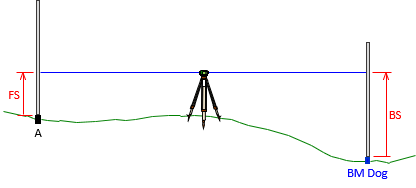## 1. Direct and Indirect Measurements

Using a measuring device on an unknown quantity to determine its value is direct measurement. The value is determined comparing the device to the quantity and reading the value from gradations or a digital display. Examples include measuring:

• a distance with a tape, Figure A-1
• an angle with a total station
• atmospheric pressure using a barometer.

Outside of applying systematic error corrections, no computations are necessary to determine the value from the measurement.Figure A-1 Distance Taping

Indirect measurements are used with computations to determine an unknown quantity. Examples include:

• elevation determination using backsight and foresight readings, Figure A-2
• spot elevations by three dimensional surveying
• lot area from angles and distances

These are typically networks: one or more types of measurements combined with computations to determine desired value(s). We will use the term in this context when discussing network adjustment in the rest of this topic.Figure A-2 Differential Leveling

Direct and indirect measurements affect how errors propagate into the quantity being determined.